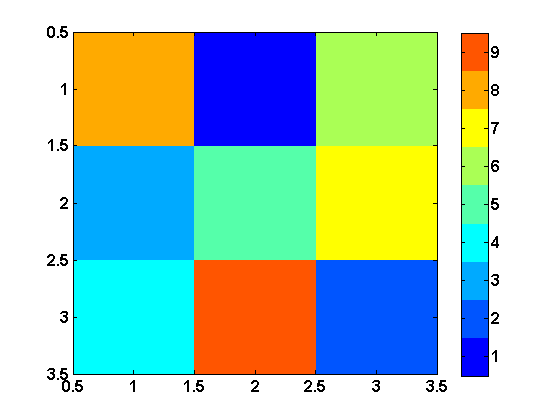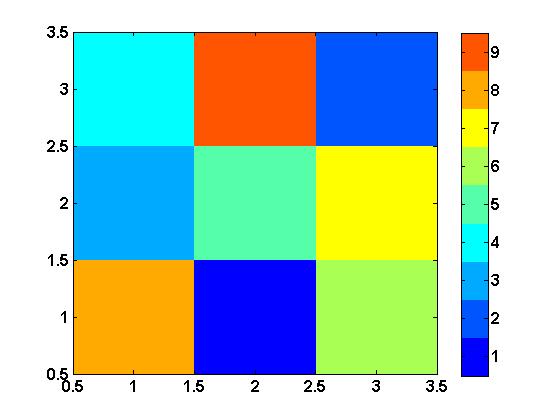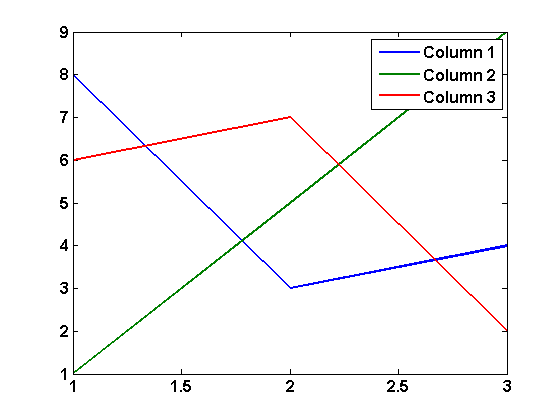## Loren on the Art of MATLABTurn ideas into MATLAB

Note

Loren on the Art of MATLAB has been retired and will not be updated.

# Indexing Terminology

Someone recently asked me to discuss X-Y versus row-column indexing.

### Two Conventions

When calling functions in MATLAB that create or reference arrays, there are two different conventions for specifying the first two dimensions of an array:

• matrix indexing, i.e., rows and columns
• Cartesian , i.e., X and Y

These different conventions get used depending on whether the array is strictly an array or when it has a probable physical interpretation in terms of Cartesian grid.

### Matrix versus Cartesian Frameworks

In MATLAB, we index into matrices using row and column indices. That is, for matrix

A = magic(3)
A =
8     1     6
3     5     7
4     9     2


I can reference the last element in the second row like this:

A(2,3)
ans =
7


or

A(2,end)
ans =
7


and to retrieve the first element of columns 1 and 3, I type this:

A(1,[1 3])
ans =
8     6


Check out this post to get a fairly complete picture of indexing in MATLAB.

### Plotting Matrices

The options xy and ij for axis allow us to control whether to view the data just the way the matrix is displayed (the (1,1) element is in the upper left corner):

colormap(jet(9))
image(A)
colorbaror we can view the image/matrix as a top-down view of a physical "map" with the y-values now increasing along the vertical axis.

axis xyIn images, the matrices column values plot vertically. In line plots, the columns are plotted vs. their indices, making columns plot horizontally.

h = plot(A);
legend(h,{'Column 1','Column 2','Column 3'})### Creating an N-Dimensional Grid

ndgrid uses matrix indexing since it is designed to suit working in any number of array dimensions.

[ii, jj] = ndgrid(1:3,1:2)
ii =
1     1
2     2
3     3
jj =
1     2
1     2
1     2


### Creating an 2- or 3-Dimensional Grid

On the other hand, mesh, interprets its first inputs in a Cartesian framework since it is used primarily for working with 2- and 3-dimensional plots, often depicting something over an area or volume.

[xx, yy] = meshgrid(1:3,1:2)
xx =
1     2     3
1     2     3
yy =
1     1     1
2     2     2


In the Remarks section of the documentation for meshgrid, you will find a discussion of this, especially with respect to inverting the first two input arguments compared to ndgrid. There is related discussion in the Description section for the function mesh.

Functions designed for two or three dimension, such as contour and quiver, usually work in Cartesian coordinates. Functions that are designed to work in N-dimensions generally work with a matrix indexing framework. Be sure to check the help for functions you use to see which convention applies.

### Thoughts?

Any ideas on what we can do, perhaps in examples or documentation (or eslewhere?) to help people out with this potential confusion? If so, please post here.

Published with MATLAB® 7.4

|